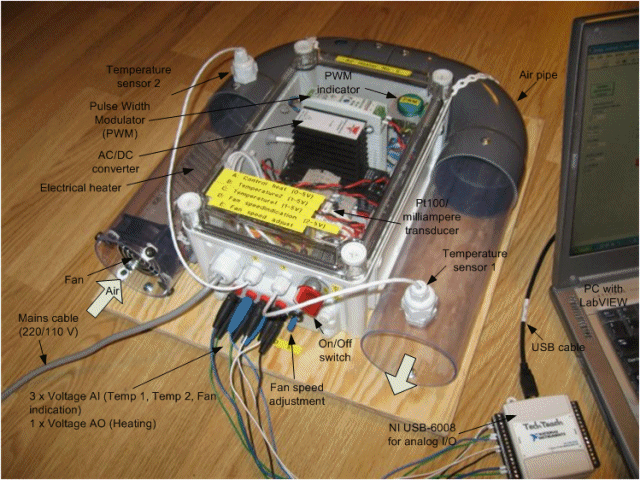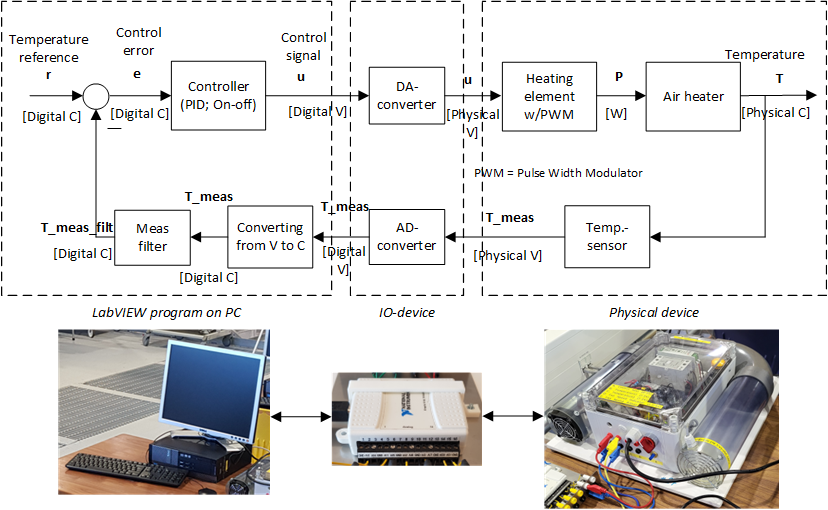# Lab Station: Air Heater

## Description of the system

Figure 1 shows an air tube with heater and temperature sensor(s). University College of South-Eastern Norway (campus Porsgrunn), has 24 copies of this lab station, being used in several control courses in both bachelor and master programmes in technology.Figure 1

## Video presenting the air heater

In the video, the home page of the air heater is erroneously presented as home.hit.no/~finnh/air_heater. The correct address is http://techteach.no/air_heater.

## Mathematical model

A mathematical model that has proven to describe quite well the dynamic behaviour of the outlet air temperature is given by the following differential equation representing "time-constant with time-delay" dynamics from control signal u to outlet temperature T:

dT/dt = [(Tenv - T) + Kh * u(t - tdelay)]/tconst    (Eq. 1)

where:

·       T [C] is the tube outlet temperature (C is Celcius). (On the real air heater T is measured with a Pt100 sensor.)

·       Tenv [C] is the environment (room) temperature.

·       u [V] is the control signal to the heater.

·       Kh [C/V] is the heater gain.

·       tconst [s] is the time-constant.

·       tdelay [s] is the time-delay representing air transportation and “sluggishness” of the heater.

The above model may be derived from mechanistic (first-principles) modeling principles, i.e. a simple energy balance of the air, if we make the idealized assumption that the tube is a so-called CSTR (continuous stirred tank reactor) with air inflow and outflow and heat transfer with the environment through the “reactor” (tube) walls, and - in addition - we include the time-delay as described above. In reality, the idealized CSTR conditions are not satisfied, but they lead to a useful model structure with parameter values that may be estimated from experimental data. (Many other stable physical processes show “time-constant with time-delay” dynamics, and such processes may be reasonably well represented with models like Eq. 1.)

In a simulator based on this model a proper initial value, Tinit, of the state variable T must defined. If you assume that the heater has been turned off for a while, you can set Tinit = Tenv.

The parameter values vary somewhat among the lab stations. However, the following values are typical and can be used (e.g. in a simulator) unless you have found other values from experiments:

·       Kh = 3.5 C/V

·       tconst = 23 s

·       tdelay = 3 s

·       Tenv = 20 deg C

## Experimental data

airheater_logfile.txt contains data from an experiment on the air heater. (The fan speed was kept constant during the experiment.) The file containes three colums of data:

• Time, t [s]
• Control signal to the heater, u [V]
• Outlet temperature, T [C].

## Block diagram of temperature control system with LabVIEW(Click on the picture for showing picture as PDF file.)

## Technical information

Each air heater consists of the following items:

1.     One plywood plate on which the devices are mounted

2.     Plastic box containing all electrical devices

3.     One plastic tube

4.     One air fan (originally a PC fan)

5.     One potensiomter (variable resistance) for manual adjustment of the voltage controlling the fan speed.

6.     One electric power cable (for connection to mains outlet, e.g. 220 V)

7.     Two temperature sensors, type Pt100, with a measurement signal converter from resistance to current (INOR miniPack-L)

8.     Precision resistors for converting temperature measurement current to voltage

9.     One heating element (coil) for electric heating of air. The coil is originally used in a shoe dryer. Power (assuming 220 VAC) is 250 W.

10.  One electrical AC-DC converter from 220 VAC to 24 VDC. Datasheet_power_supply.pdf

11.  One Pulse-width modulator (PWM): Carlo Gavazzi RN F23V30. Datasheet_ssr_pwm.pdf

## Publication

·       F. Haugen, Fjelddalen E, Edgar T., Dunia R., Demonstrating PID Control Principles using an Air Heater and LabVIEW,

Updated 5th May 2023 by Finn Aakre Haugen. E-mail Finn.Haugen@usn.no.